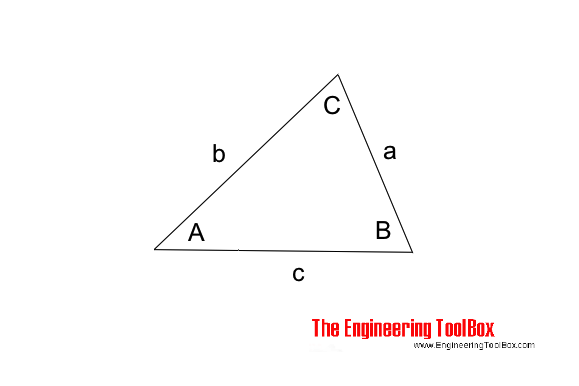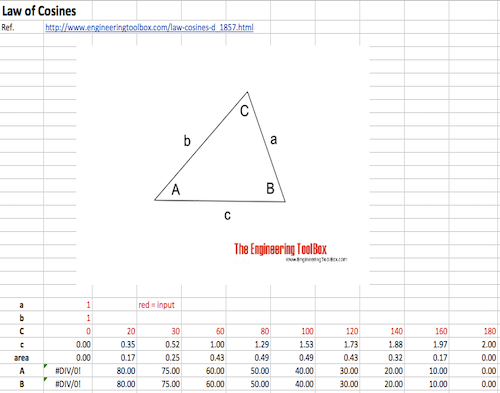Engineering ToolBox - Resources, Tools and Basic Information for Engineering and Design of Technical Applications!

# Law of Cosines

## One side of a triangle when the opposite angle and two sides are known.

The "Law of Cosines" can be used to calculate one side of a triangle when the angle opposite and the other two sides are known.The "Law of Cosines" can be expressed as

$$c^2 = a^2 + b^2 - 2 a b cos C \tag{1}$$

where

a, b and c = length of triangle sides (m, ft ..)

C = angle opposite side c (degrees)

### Example - Calculate Side in Triangle

If side a = 1 m, side b = 1.3 m and angle C = 60 degrees - the side c can be calculated by modifying eq. 1 to

$$c = \sqrt{ (1 m)^2 + (1.3 m)^2 - 2 (1 m) (1.3 m) cos (60 degrees) } = \underline{1.18} m$$

### Law of Cosines Calculator

The generic calculator below can used to calculate the side c:

a (m, ft ....)

b (m, ft ....)

C (degrees)

### Law of Cosines - Excel Template## Related Topics

• ### Mathematics

Mathematical rules and laws - numbers, areas, volumes, exponents, trigonometric functions and more.

## Related Documents

• ### AC - Active, Reactive and Apparent Power

Real, imaginary and apparent power in AC circuits.
• ### Exponents - Powers and Roots

The laws of fractional and integer exponents.
• ### Factorials

The product of all positive integers.
• ### Fractions

Law of fractions
• ### Hyperbolic Functions

Exponential functions related to the hyperbola.
• ### Law of Sines

Calculate the angles in a generic triangle.
• ### Law of Tangents

Triangles and law of tangents.
• ### Pythagorean Theorem

Verifying square corners.
• ### Right Angled Triangle

Right angled triangle equations.
• ### Standard Differentials and Integrals

Equations for differentials and integrals.
• ### Three-Phase Electrtical Motors - Power Factor vs. Inductive Load

Inductive loads and power factors with electrical three-phase motors.
• ### Three-Phase Power Equations

Electrical 3-phase equations.
• ### Triangle

Triangle analytical geometry.
• ### Trigonometric Functions

Sine, cosine and tangent - the natural trigonometric functions.

Online vector calculator - add vectors with different magnitude and direction - like forces, velocities and more.
• ### Wire Rope Slings

Sling angles and influence on capacity.

## Engineering ToolBox - SketchUp Extension - Online 3D modeling!

Add standard and customized parametric components - like flange beams, lumbers, piping, stairs and more - to your Sketchup model with the Engineering ToolBox - SketchUp Extension - enabled for use with older versions of the amazing SketchUp Make and the newer "up to date" SketchUp Pro . Add the Engineering ToolBox extension to your SketchUp Make/Pro from the Extension Warehouse !

We don't collect information from our users. More about

## Citation

• The Engineering ToolBox (2013). Law of Cosines. [online] Available at: https://www.engineeringtoolbox.com/law-cosines-d_1857.html [Accessed Day Month Year].

Modify the access date according your visit.

9.19.12